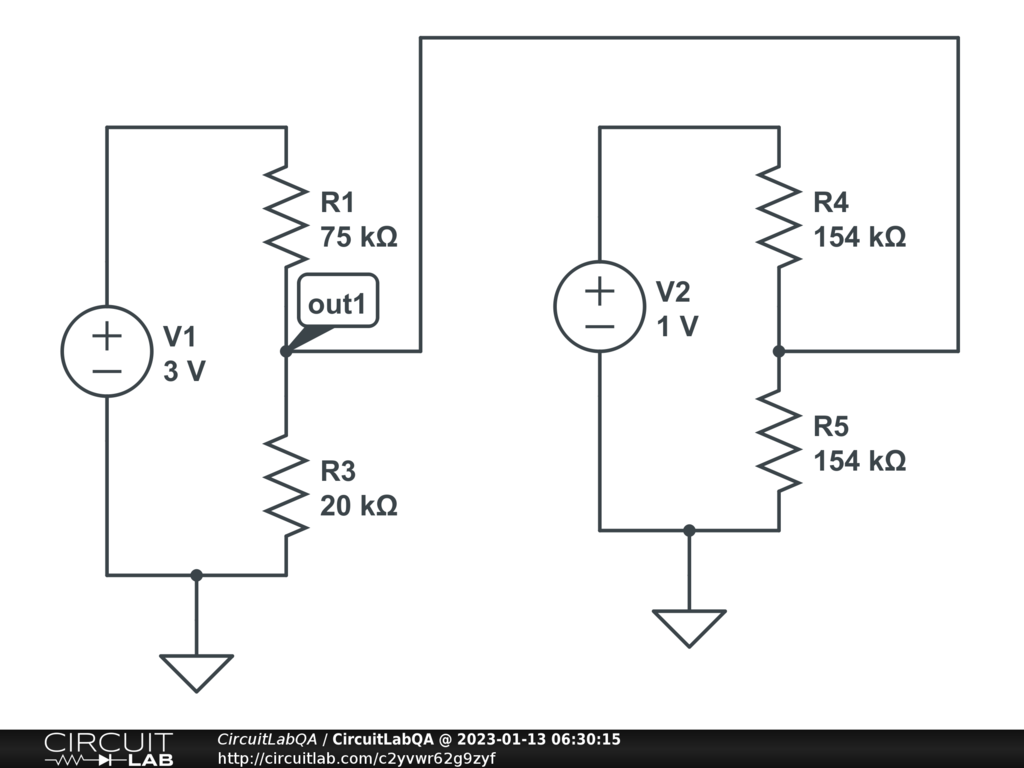## Voltage dividerHi, May i know what is formula to calculate the voltage divider for this circuit ? by ashrof January 13, 2023 It is NOT a voltage divider. To be a voltage divider, the same current should flow through the two resistors. Here, some current from R1 is allowed to escape toward R5. The equations can be: Loop through V1: 3 = iR1 R1 + iR3R3 Loop through V2: 1 = iR4 R4 + iR5R5 Loop through R2, cable without resistor, R5, ground: 0 = -iR2R2 + iR5 R5 (The - sign is needed since we travel the loop backward across R3) And the fourth equation is supplied by the sum of current at the nodes with the wire without resistor: iR1 - iR3 = iwire = iR5 - iR4 Solve 4 equations with 4 unknowns. iwire has to be zero for the voltage divider formula to be applicable. (or close to 0 to get an good approximation, as when it is 10 000 less than iR1). by vanderghast January 13, 2023 Thanks by ashrof January 13, 2023 Add comment... Please sign in or create an account to comment.

CircuitLab's Q&A site is a FREE questions and answers forum for electronics and electrical engineering students, hobbyists, and professionals.

We encourage you to use our built-in schematic & simulation software to add more detail to your questions and answers.

Acceptable Questions:

• Concept or theory questions
• Practical engineering questions
• “Homework” questions
• Software/hardware intersection
• Best practices
• Design choices & component selection
• Troubleshooting

Unacceptable Questions:

• Non-English language content
• Non-question discussion
• Non-electronics questions
• Vendor-specific topics
• Pure software questions
• CircuitLab software support

Please respect that there are both seasoned experts and total newbies here: please be nice, be constructive, and be specific!